# 机器学习：支持向量机如何应用于交易

4 三月 2014, 10:35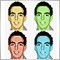1
3 332

### 何为“支持向量机”？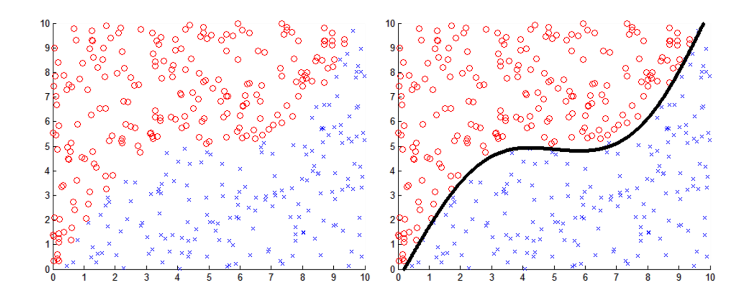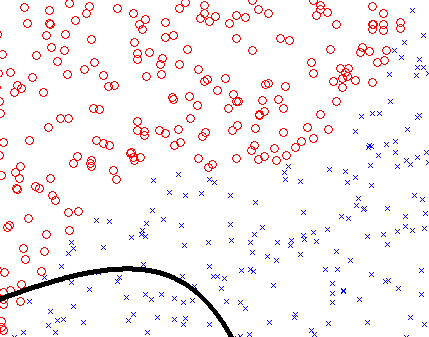### 支持向量机的工作原理示例：这是 Schnick 吗？

• 身高
• 体重
• 肢体数量
• 眼睛数量
• 前肢长度
• 平均奔跑速度
• 交配鸣叫频率

• 数据必须包含 schnick 这一动物的案例
• 数据必须包含非 schnick 动物的案例

### 支持向量机到底工作了吗？

N_legs 8 10
N_eyes 3 4
L_arm [mm] 400 450
av_speed [m/s] 2 2.5
f_call [Hz] 11000 15000

```//+------------------------------------------------------------------+
//| This function takes the observation properties of the observed
//| animal and based on the criteria we have chosen, returns true/false whether it is a schnick
//+------------------------------------------------------------------+
bool isItASchnick(double height,double weight,double N_legs,double N_eyes,double L_arm,double av_speed,double f_call)
{
if(height   < 1000  || height   > 1100)  return(false);   // If the height is outside the parameters > return(false)
if(weight   < 40    || weight   > 50)    return(false);   // If the weight is outside the parameters > return(false)
if(N_legs   < 8     || N_legs   > 10)    return(false);   // If the N_Legs is outside the parameters > return(false)
if(N_eyes   < 3     || N_eyes   > 4)     return(false);   // If the N_eyes is outside the parameters > return(false)
if(L_arm    < 400   || L_arm    > 450)   return(false);   // If the L_arm  is outside the parameters > return(false)
if(av_speed < 2     || av_speed > 2.5)   return(false);   // If the av_speed is outside the parameters > return(false)
if(f_call   < 11000 || f_call   > 15000) return(false);   // If the f_call is outside the parameters > return(false)
return(true);                                             // Otherwise > return(true)
}```

```//+------------------------------------------------------------------+
//| This function takes an empty double array and an empty boolean array,
//| and generates the inputs/outputs to be used for training the SVM
//+------------------------------------------------------------------+
void genTrainingData(double &inputs[],bool &outputs[],int N)
{
double in[];                    // Creates an empty double array to be used for temporarily storing the inputs generated
ArrayResize(in,N_Inputs);       // Resize the in[] array to N_Inputs
ArrayResize(inputs,N*N_Inputs); // Resize the inputs[] array to have a size of N*N_Inputs
ArrayResize(outputs,N);         // Resize the outputs[] array to have a size of N
for(int i=0;i<N;i++)
{
in=    randBetween(980,1120);      // Random input generated for height
in=    randBetween(38,52);         // Random input generated for weight
in=    randBetween(7,11);          // Random input generated for N_legs
in=    randBetween(3,4.2);         // Random input generated for N_eyes
in=    randBetween(380,450);       // Random input generated for L_arms
in=    randBetween(2,2.6);         // Random input generated for av_speed
in=    randBetween(10500,15500);   // Random input generated for f_call
ArrayCopy(inputs,in,i*N_Inputs,0,N_Inputs);                         // Copy the new random inputs generated into the training input array
outputs[i]=isItASchnick(in,in,in,in,in,in,in); // Assess the random inputs and determine if it is a schnick
}
}
//+------------------------------------------------------------------+
//| This function is used to create a random value between t1 and t2
//+------------------------------------------------------------------+
double randBetween(double t1,double t2)
{
return((t2-t1)*((double)MathRand()/(double)32767)+t1);
}
```

```void OnStart()
{
double inputs[];              // Empty double array to be used for creating training inputs
bool   outputs[];             // Empty bool array to be used for creating training inputs
int    N_TrainingPoints=5000; // Defines the number of training samples to be generated
int    N_TestPoints=5000;     // Defines the number of samples to be used when testing

genTrainingData(inputs,outputs,N_TrainingPoints); //Generates the inputs and outputs to be used for training the SVM

int handle1=initSVMachine();             // Initializes a new support vector machine and returns a handle
setInputs(handle1,inputs,7);             // Passes the inputs (without errors) to the support vector machine
setOutputs(handle1,outputs);             // Passes the outputs (without errors) to the support vector machine
setParameter(handle1,OP_TOLERANCE,0.05); // Sets the error tolerance parameter to <5%
training(handle1);                       // Trains the support vector machine using the inputs/outputs passed
}```

```//+------------------------------------------------------------------+
//| This function takes the handle for the trained SVM and tests how
//| successful it is at classifying new random inputs
//+------------------------------------------------------------------+
double testSVM(int handle,int N)
{
double in[];
int atrue=0;
int afalse=0;
int N_correct=0;
bool Predicted_Output;
bool Actual_Output;
ArrayResize(in,N_Inputs);
for(int i=0;i<N;i++)
{
in=    randBetween(980,1120);      // Random input generated for height
in=    randBetween(38,52);         // Random input generated for weight
in=    randBetween(7,11);          // Random input generated for N_legs
in=    randBetween(3,4.2);         // Random input generated for N_eyes
in=    randBetween(380,450);       // Random input generated for L_arms
in=    randBetween(2,2.6);         // Random input generated for av_speed
in=    randBetween(10500,15500);   // Random input generated for f_call
Actual_Output=isItASchnick(in,in,in,in,in,in,in); // Uses the isItASchnick fcn to determine the actual desired output
Predicted_Output=classify(handle,in);                                  // Uses the trained SVM to return the predicted output.
if(Actual_Output==Predicted_Output)
{
N_correct++;   // This statement keeps count of the number of times the predicted output is correct.
}
}

return(100*((double)N_correct/(double)N));   // Returns the accuracy of the trained SVM as a percentage
}```

### 为何支持向量机如此有用？

```//+------------------------------------------------------------------+
//| This function takes the correct training inputs and outputs generated
//| and inserts N random errors into the data
//+------------------------------------------------------------------+
void insertRandomErrors(double &inputs[],bool &outputs[],int N)
{
int    nTrainingPoints=ArraySize(outputs); // Calculates the number of training points
int    index;                              // Creates new integer 'index'
bool   randomOutput;                       // Creates new bool 'randomOutput'
double in[];                               // Creates an empty double array to be used for temporarily storing the inputs generated
ArrayResize(in,N_Inputs);                  // Resize the in[] array to N_Inputs
for(int i=0;i<N;i++)
{
in=    randBetween(980,1120);        // Random input generated for height
in=    randBetween(38,52);           // Random input generated for weight
in=    randBetween(7,11);            // Random input generated for N_legs
in=    randBetween(3,4.2);           // Random input generated for N_eyes
in=    randBetween(380,450);         // Random input generated for L_arms
in=    randBetween(2,2.6);           // Random input generated for av_speed
in=    randBetween(10500,15500);     // Random input generated for f_call

index=(int)MathRound(randBetween(0,nTrainingPoints-1)); // Randomly chooses one of the training inputs to insert an error
if(randBetween(0,1)>0.5) randomOutput=true;             // Generates a random boolean output to be used to create an error
else                     randomOutput=false;

ArrayCopy(inputs,in,index*N_Inputs,0,N_Inputs);         // Copy the new random inputs generated into the training input array
outputs[index]=randomOutput;                            // Copy the new random output generated into the training output array
}
}```

```void OnStart()
{
double inputs[];              // Empty double array to be used for creating training inputs
bool   outputs[];             // Empty bool array to be used for creating training inputs
int    N_TrainingPoints=5000; // Defines the number of training samples to be generated
int    N_TestPoints=5000;     // Defines the number of samples to be used when testing

genTrainingData(inputs,outputs,N_TrainingPoints); // Generates the inputs and outputs to be used for training the svm

int handle1=initSVMachine();             // Initializes a new support vector machine and returns a handle
setInputs(handle1,inputs,7);             // Passes the inputs (without errors) to the support vector machine
setOutputs(handle1,outputs);             // Passes the outputs (without errors) to the support vector machine
setParameter(handle1,OP_TOLERANCE,0.05); // Sets the error tolerance parameter to <5%
training(handle1);                       // Trains the support vector machine using the inputs/outputs passed

insertRandomErrors(inputs,outputs,500);  // Takes the original inputs/outputs generated and adds random errors to the data

int handle2=initSVMachine();             // Initializes a new support vector machine and returns a handle
setInputs(handle2,inputs,7);             // Passes the inputs (with errors) to the support vector machine
setOutputs(handle2,outputs);             // Passes the outputs (with errors) to the support vector machine
setParameter(handle2,OP_TOLERANCE,0.05); // Sets the error tolerance parameter to <5%
training(handle2);                       // Trains the support vector machine using the inputs/outputs passed

double t1=testSVM(handle1,N_TestPoints); // Tests the accuracy of the trained support vector machine and saves it to t1
double t2=testSVM(handle2,N_TestPoints); // Tests the accuracy of the trained support vector machine and saves it to t2

Print("The SVM accuracy is ",NormalizeDouble(t1,2),"% (using training inputs/outputs without errors)");
Print("The SVM accuracy is ",NormalizeDouble(t2,2),"% (using training inputs/outputs with errors)");
deinitSVMachine();                       // Cleans up all of the memory used in generating the SVM to avoid memory leak
}```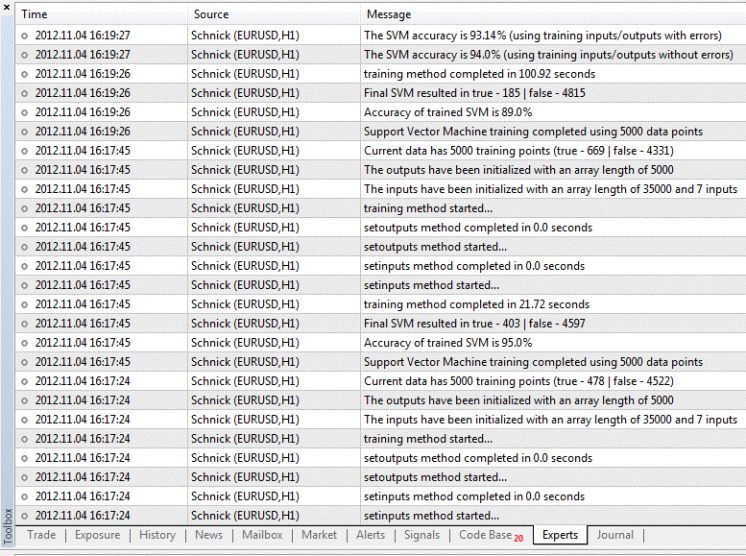### 演示版本

“代码库”中可下载上述代码的完整版本，但是只有在您从“应用商店”购买了完整版本的“支持向量机学习工具”的情况下，才能在您的终端运行此脚本。如果您只是下载了此工具的演示版，则仅限于通过策略测试程序使用。为了能用此工具的演示版测试 "Schnick" 代码，我将此脚本改写成了可利用策略测试程序配置的“EA 交易”。两种版本的代码，都可以使用下述链接下载：

• 完整版 - 使用在 MetaTrader 5 终端配置的脚本（要求购买“支持向量机学习工具”版本）

• 演示版 - 使用在 MetaTrader 5 策略测试程序中配置的 EA 交易（仅要求“支持向量机学习工具”的演示版本）

### 支持向量机如何应用于“应用市场”？

1. 监控数个指标
2. 找出每一个指标与潜在成功交易相关联的条件
3. 观察每一个指标，并在他们全部（或大部分）产生交易信号时进行评估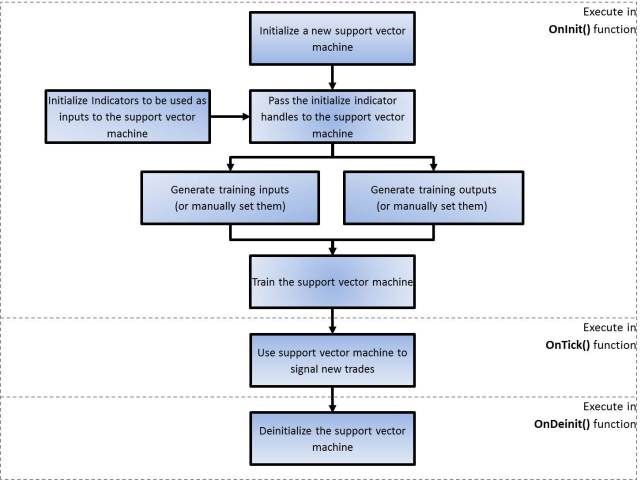### 如何生成训练输入？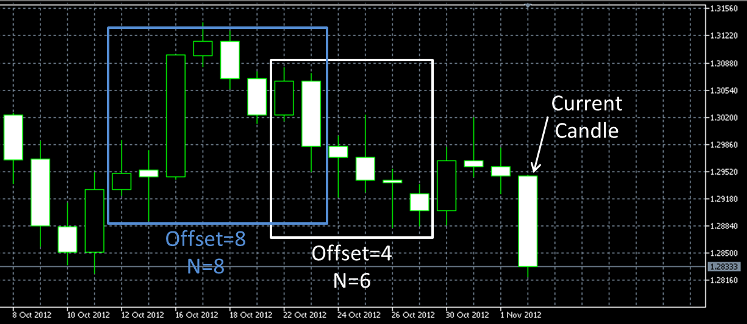### 选择输入时要考虑的事项

1. 您可以创建一个新的、使用 MACD 指标过去 5 个柱的自定义指标，以将趋势作为一个单一值计算。之后，可将这个自定义指标作为一个单一输入传递给支持向量机，或者

2. 您可以将支持向量机中 MACD 指标的过去 5 个柱作为 5 个独立的输入使用。方法则是初始化 MACD 指标的 5 个不同实例。每个指标均可利用于当前柱的某个不同偏移进行初始化。之后，就可以将来自不同指标的 5 个句柄传递给支持向量机。要注意的是，第 2 种选项很容易导致 EA 交易执行时间的延长。您的输入越多，成功训练所需的时间就越长。

### EA 交易中实施支持向量机

1. 创建两个使用 svMachineTool 库的新的支持向量机。一个设置用于生成新“买入”交易信号，另一个则设置用于生成新“卖出”交易信号。

2. 有 7 个标准指标完成初始化，而每一个指标的句柄均存储于一个整数数组中（注意：上述指标的任何组合均可用作输入，只是需要将其以单一整数数组的形式传递给支持向量机。）

3. 指标句柄的数组被传递至新的支持向量机。

4. 利用指标句柄数组及其它参数，历史价格数据被用于生成准确的输入与输出，以供训练支持向量机使用。

5. 一旦所有的输入与输出均生成完毕，则两台支持向量机亦训练完成。

6. EA 中的训练支持向量机用于生成新的“买入”与“卖出”交易信号。生成新的“买入”或“卖出”交易信号后，交易会随着手动止损与获利订单建仓。

```#property copyright "Copyright 2011, MetaQuotes Software Corp."
#property version   "1.00"

#property indicator_buffers 7

//+---------Support Vector Machine Learning Tool Functions-----------+
//| The following #import statement imports all of the support vector
//| machine learning tool functions into the EA for use. Please note, if
//| you do not import the functions here, the compiler will not let you
//| use any of the functions
//+------------------------------------------------------------------+
#import "svMachineTool.ex5"
enum ENUM_OPTION {OP_MEMORY,OP_MAXCYCLES,OP_TOLERANCE};
int  initSVMachine(void);
void setIndicatorHandles(int handle,int &indicatorHandles[],int offset,int N);
void setParameter(int handle,ENUM_OPTION option,double value);
bool genInputs(int handle);
bool setInputs(int handle,double &Inputs[],int nInputs);
bool setOutputs(int handle,bool &Outputs[]);
bool training(int handle);
bool classify(int handle);
bool classify(int handle,int offset);
bool classify(int handle,double &iput[]);
void  deinitSVMachine(void);
#import

//+-----------------------Input Variables----------------------------+
input int            takeProfit=100;      // TakeProfit level measured in pips
input int            stopLoss=150;        // StopLoss level measured in pips
input double         hours=6;             // The maximum hypothetical trade duration for calculating training outputs.
input double         risk_exp=5;          // Maximum simultaneous order exposure to the market
input double         Tolerance_Value=0.1; // Error Tolerance value for training the SVM (default is 10%)
input int            N_DataPoints=100;    // The number of training points to generate and use.

//+---------------------Indicator Variables--------------------------+
//| Only the default indicator variables have been used here. I
//| recommend you play with these values to see if you get any
//| better performance with your EA.
//+------------------------------------------------------------------+
int bears_period=13;
int bulls_period=13;
int ATR_period=13;
int mom_period=13;
int MACD_fast_period=12;
int MACD_slow_period=26;
int MACD_signal_period=9;
int Stoch_Kperiod=5;
int Stoch_Dperiod=3;
int Stoch_slowing=3;
int Force_period=13;

int         tickets[];
bool        Opn_B,Opn_S;
datetime    New_Time;
int         handleB,handleS;
double      Vol=1;
//+------------------------------------------------------------------+
//|                                                                  |
//+------------------------------------------------------------------+
int OnInit()
{
New_Time=0;
int handles[];ArrayResize(handles,7);
//+------------------------------------------------------------------+
//| The following statements are used to initialize the indicators to be used for the support
//| vector machine. The handles returned are stored to an int[] array. I have used standard
//| indicators in this case however, you can also you custom indicators if desired
//+------------------------------------------------------------------+
handles=iBearsPower(Symbol(),0,bears_period);
handles=iBullsPower(Symbol(),0,bulls_period);
handles=iATR(Symbol(),0,ATR_period);
handles=iMomentum(Symbol(),0,mom_period,PRICE_TYPICAL);
handles=iMACD(Symbol(),0,MACD_fast_period,MACD_slow_period,MACD_signal_period,PRICE_TYPICAL);
handles=iStochastic(Symbol(),0,Stoch_Kperiod,Stoch_Dperiod,Stoch_slowing,MODE_SMA,STO_LOWHIGH);
handles=iForce(Symbol(),0,Force_period,MODE_SMA,VOLUME_TICK);

//----------Initialize, Setup and Training of the Buy-Signal support vector machine----------
handleB=initSVMachine();                             // Initializes a new SVM and stores the handle to 'handleB'
setIndicatorHandles(handleB,handles,0,N_DataPoints); // Passes the initialized indicators to the SVM with the desired offset
// and number of data points
setParameter(handleB,OP_TOLERANCE,Tolerance_Value);  // Sets the maximum error tolerance for SVM training
genInputs(handleB);                                  // Generates inputs using the initialized indicators
genOutputs(handleB,BUY,stopLoss,takeProfit,hours);   // Generates the outputs based on the desired parameters for taking hypothetical trades

//----------Initialize, Setup and Training of the Sell-Signal support vector machine----------
handleS=initSVMachine();                             // Initializes a new SVM and stores the handle to 'handleS'
setIndicatorHandles(handleS,handles,0,N_DataPoints); // Passes the initialized indicators to the SVM with the desired offset
// and number of data points
setParameter(handleS,OP_TOLERANCE,Tolerance_Value);  // Sets the maximum error tolerance for SVM training
genInputs(handleS);                                  // Generates inputs using the initialized indicators
genOutputs(handleS,SELL,stopLoss,takeProfit,hours);  // Generates the outputs based on the desired parameters for taking hypothetical trades
//----------
training(handleB);   // Executes training on the Buy-Signal support vector machine
training(handleS);   // Executes training on the Sell-Signal support vector machine
return(0);
}```

### 高级支持向量机交易

| A1 | B1 | C1 | A2 | B2 | C2 | A3 | B3 | C3 | A4 | B4 | C4 | A5 | B5 | C5 |

• 生成自己的输入与输出时，要确保数组的长度与您传入的值相匹配。如不匹配，则会生成一个错误，提醒您有背离。示例：如果我们已传入 N_Inputs=3、而输入是一个长度为 16 的数组，则会出现一个错误（因为 N_inputs 为 3，则意味着任何输入数组的长度都要是 3 的倍数）。同样，还要确保输入集的数量与您传递的输出数量相等。同样，如果 N_Inputs=3、输入长度为 15、而输出长度为 6，则会出现另一个错误（因为您有 5 组输入和 6 组输出）。

• 尽力确保训练输出中有足够的变量。示例：如果您传入 100 个训练点（意味着输出数组长度为 100），而且所有值只有一个为 true（其他均为 false），那么，true 与 false 之间的区别并不足够。如此则容易导致支持向量机训练非常快，但最终结果却非常差。而训练集越多样化，支持向量机往往也会更有效。

schnick.mq5 (10.8 KB)
schnick_demo.mq5 (11.39 KB)| 26 5月 2015 在 08:23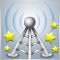MQL5 信号的优势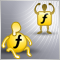通过辅助指标减少内存消耗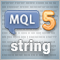MQL5 编程基础：字符串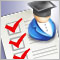购买交易机器人前如何进行测试